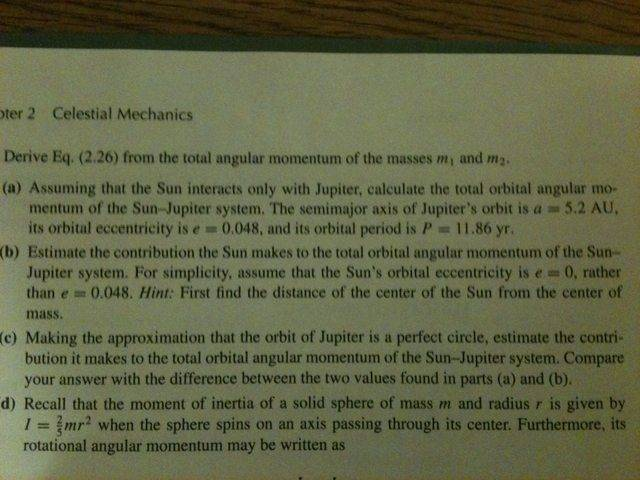# Orbital Angular Momentum of the Sun-Jupiter System

jinksys

## Homework StatementIn part A I know that I must use L = mu*Sqrt[GMa(1-e^2)], but for the variable 'a' do I use the semi-major axis of jupiter or the semi-major axis of the reduced mass?

## The Attempt at a Solution

zorro
I don't know how you got that equation. This question does not require reduced mass concept. The Sun is stationary and Jupiter revolves around it.

Use L=mvr
substitute v from the equation T=2(pi)r/v
Now the r is actually the average of the radius at apogee and perigee.
i.e. r=(ra + rp)/2
Use properties of ellipse to find the unknown from the above equation.
If you have the mass of Jupiter you are done with the question.

Staff Emeritus
I don't know how you got that equation. This question does not require reduced mass concept. The Sun is stationary and Jupiter revolves around it.
This is wrong. The question is rather explicitly asking the student to consider the problem of a non-circular orbit of an object with enough mass that the simplistic Keplerian approach is no longer valid.

In part A I know that I must use L = mu*Sqrt[GMa(1-e^2)], but for the variable 'a' do I use the semi-major axis of jupiter or the semi-major axis of the reduced mass?
How do you know that that is the equation you need to use?

Hint: Depending on what you mean by the "semi major axis of Jupiter", this is also wrong.

The question is a bit sloppy in that it doesn't say what that 5.2 AU means. The usual meaning is in terms of Jupiter's orbit about the Sun rather than Jupiter's orbit about the barycenter.

zorro
This is wrong. The question is rather explicitly asking the student to consider the problem of a non-circular orbit of an object with enough mass that the simplistic Keplerian approach is no longer valid.

Can you explain me what is wrong?
Is there any problem with averaging the radius? Does the Sun + Jupiter revolve around their common centre of mass?

Staff Emeritus
Can you explain me what is wrong?
Is there any problem with averaging the radius?
Yes,there is. You can't average the the radius and then assume as you said that "T=2(pi)r/v".

Does the Sun + Jupiter revolve around their common centre of mass?
Yes, they do.

zorro
I think I have misunderstood something.
This is a question in my book-
A planet is revolving around the sun. Its distance from the sun at apogee is Ra and that at perigee is Rp. The mass of the planet and sun is m and M resp, T is the time period of revolution of planet round the sun. What is the relation between T,M, Ra and Rp?

The answer given is T2 = π2(Ra + Rp)3/2GM, which is obtained by averaging the radius.

Is the answer given wrong? If yes then what laws should we use to find the relation?